# Samacheer Kalvi 7th Maths Solutions Term 1 Chapter 6 Information Processing Ex 6.2

Students can Download Maths Chapter 6 Information Processing Ex 6.2 Questions and Answers, Notes Pdf, Samacheer Kalvi 7th Maths Book Solutions Guide Pdf helps you to revise the complete Tamilnadu State Board New Syllabus and score more marks in your examinations.

## Tamilnadu Samacheer Kalvi 7th Maths Solutions Term 1 Chapter 6 Information Processing Ex 6.2

Miscellaneous Practice Problems

Question 1.
Make a model of a fish using the given tetromino shapes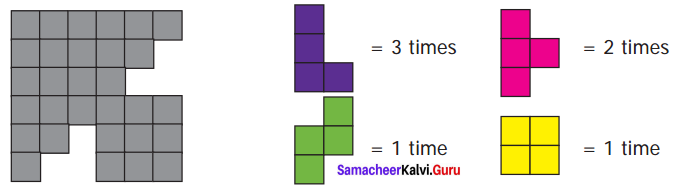Complete the given rectangle using the given tetromino shapes.
Solution: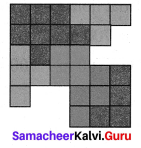Question 2.
Complete the given rectangle using the given tetromino shapes.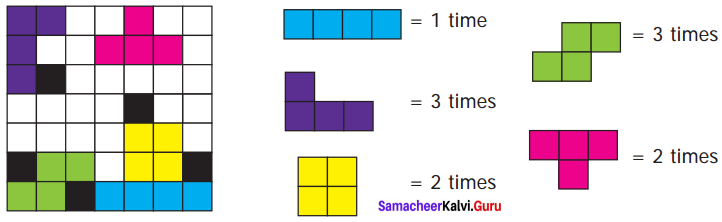Solution: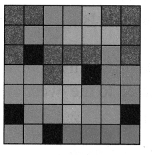Question 3.
Shade the figure completely, by using five Tetromino shapes only once.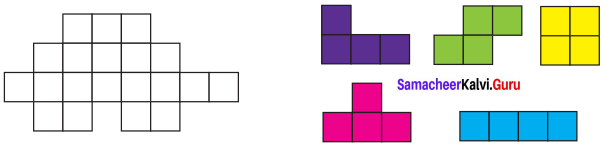Solution: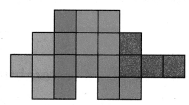Question 4.
Using the given tetrominoes with numbers on it complete the 4 × 4 magic square?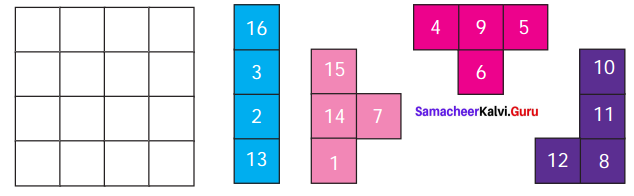Solution: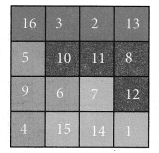Question 5.
Find the shortest route to Vivekanandar Memorial Hall from the Mandapam using the given map.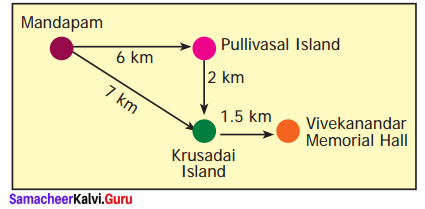Solution:
Possible routes from Mandapam to Vivekandar Memorial are

route 1:
(a) Mandapam ➝ Pullivasal Island ➝ Krusadai Island ➝ Vivekanandar Memorial Hall.
Distance = 6 Km + 2 Km + 1.5 Km = 9.5 Km

route 2:
(b) Mandapam ➝ Krusadai Island ➝ Vivekanandar Memorial Hall.
Distance = 7 Km + 1.5 Km = 8.5 Km
8.5 km < 9.5 km
∴ Shortest route : Mandapam ➝ Krusadai Island ➝ Vivekanandar Memorial Hall.

Challenge Problems

Question 6.
Fill in 4 × 10 rectangle completely, using all the five tetrominoes twice.
Solution: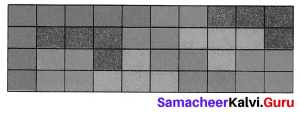(more possible ways are there)

Question 7.
Fill in 8 × 5 rectangle completely, using all the five tetrominoes twice.
Solution: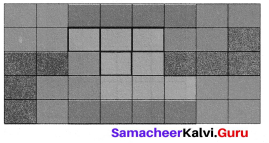(more possible way are there)

Question 8.
Observe the picture and answer the following.
(i) Find all the possible routes from A to D.
(ii) Find the shortest distance between E and C.
(iii) Find all the possible routes between B and F with distance. Mention the shortest route.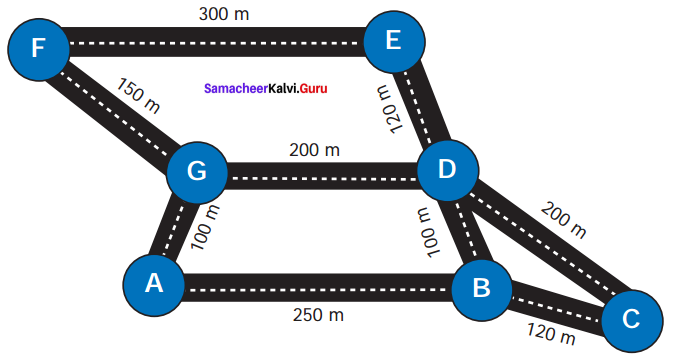Solution:
(i) All possible routes from A to D are :
(a) A ➝ G ➝ F ➝ E ➝ D
(b) A ➝ G ➝ D
(c) A ➝ B ➝ C ➝ D
(d) A ➝ B ➝ D

(ii) Distance between E and C are
(a) Route 1: E ➝ D ➝ C
Distance: 120 m + 200 m = 320 m.
(b) Route 2: E ➝ D ➝ B ➝ C
Distance = 120 + 100 m + 120 m
= 340 m.
∴ Shortest distance is 320 m.

(iii) All possible routes between B and F are :
(a) Route 1: B ➝ A ➝ G ➝ F
Distance = 250 m + 100 m + 150 m = 600 m.

(b) Route 2: 2 ➝ D ➝ E ➝ F
Distance = 100 m + 120 m + 300 m
= 520 m.

(c) Route 3: B ➝ D ➝ G ➝ F
Distance = 100 m + 200 m + 150 m
= 450 m.

(d) Route 4: B ➝ C ➝ D ➝ E ➝ F
Distance = 120 m + 200 m + 120 m + 300 m
= 740 m.
We find that Route 3 is shortest.
ie B ➝ D ➝ G ➝ F is the shortest route.